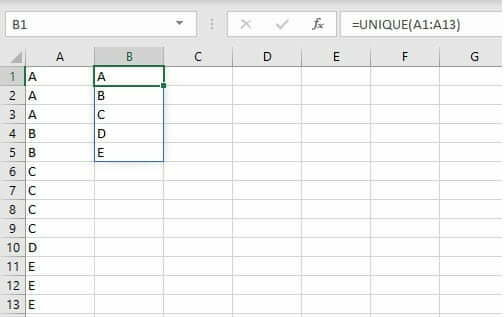## Excel – Unique & Transpose

I use Excel (O365) for data manipulation on a regular basis, as such I tend to have a need to use a wide range of formulas to process and manipulate that data. In this post, I wanted to discuss two (2) of the most useful formulas which I commonly use. The first formula is unique, this returns a list of unique values from the range specified. The formula is: =unique(range, by_column, Occurs_Only_Once) In the above formula, only the range is […]